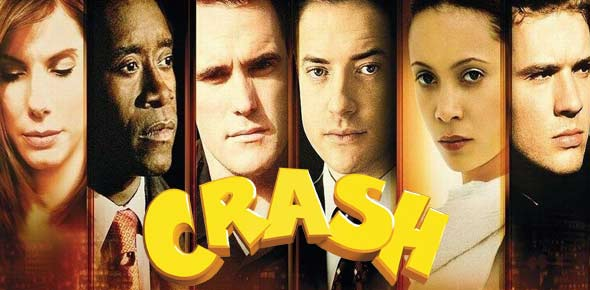# Quant Crash Course - Quiz 1

11 Questions | Total Attempts: 21SettingsThis quiz tests you on material covered within Part 1(a, b &c): understanding the distribution and its various elements; volatility and correlation; convexity; specific model terminology; options.

Related Topics
• 1.
Risk management can stop you from making losses.
• A.

True

• B.

False

• 2.
Which of the following may be assessed from a distribution of credit scores? I.   Effectiveness  of a risk manager II.  Behavior of the risk portfolio over a period of time III. Dissection of the risk portfolio across products
• A.

I &II

• B.

I&III

• C.

II&III

• D.

I, II & III

• E.

None of the above

• 3.
Which of the following is not a term used to describe the volatility in a distribution?
• A.

Sigma

• B.

Sigma a,b

• C.

Standard Deviation

• D.

Diffusion

• E.

Dispersion

• 4.
• A.

Volatility derived from historical market prices

• B.

Volatility derived from empirical data

• C.

Volatility for which model price equals market price

• D.

Volatility at which you are willing to trade the security

• E.

None of the above

• 5.
Volatility is not a static measure over time and can change rapidly from one point in time to the next.
• A.

True

• B.

False

• 6.
The interest rate differential between a local currency and a foreign currency is approximately
• A.

Equal to the expected differential between the local inflation rate and the foreign interest rate

• B.

Equal to the expected differential between the local interest rate and the foreign inflation rate

• C.

Equal to the differential between the forward exchange rate and the foreign interest rate

• D.

Equal to the differential between the local interest rate and the spot FX rate

• E.

Equal to the expected change in spot FX rates

• 7.
Why is correlation considered an enemy to the risk manager?
• A.

Because common risk models assume stable correlations whereas underlying correlations remain unchanged over time

• B.

Because common risk models assume stable correlations whereas underlying correlations change over time

• C.

Because common risk models assume unstable correlations whereas underlying correlations remain unchanged over time

• D.

Because common risk models assume unstable correlations whereas underlying correlations change over time

• E.

None of the above

• 8.
The underlying trend in model terms is known as:
• A.

Diffusion

• B.

Distribution

• C.

Drift

• D.

Dispersion

• E.

Deviation

• 9.
Convexity
• A.

Is the change in price due to a given change in the interest rate

• B.

Is the change in duration due to a given change in the price

• C.

Is the change in option value due to a given change in the price of a bond

• D.

Means that the price rises by a larger amount when interest rates fall by a given Δ% than it declines when interest rates rise by that Δ%

• E.

Means that the price rises by a smaller amount when interest rates fall by a given Δ% than it declines when interest rates rise by that Δ%

• 10.
A put option gives the buyer of the contract the right to:
• A.

Buy the asset at a predetermined price at a predetermined point in the future

• B.

Buy the asset at the prevailing price at a predetermined point in the future

• C.

Sell the asset at a predetermined price at a predetermined point in the future

• D.

Sell the asset at the prevailing price at a predetermined point in the future

• E.

Sell the asset immediately when the price of the underlying asset rises

• 11.
A put option on a bond is more valuable when:
• A.

Volatility is high

• B.

Volatility is low

• C.

Volatility has no impact on the value of the option